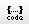PL/SQL 프로시저 생성 오류 질문드립니다. 0 1 212

by agb9380 [2021.07.24 01:24:16]

프로시져 생성 오류가 발생하여 질문드립니다.. 어디가 오류인지 모르겠습니다.. 오류select 해보니,

"PLS-00103: 심볼 "EXCEPTION"를 만났습니다 다음 중 하나가 기대될 때:
( begin case declare else end exit for goto if loop mod null
pragma raise return select update when while with <식별자>
<큰 따옴표로 구분된 식별자> <바인드 변수> <<
continue close current delete fetch lock insert open rollback
savepoint set sql execute commit forall merge pipe purge
json_exists json_value json_query json_object json_array
"

이렇게 뜹니다..

create or replace procedure test_transfer
(
V_WITHDRAW_CODE CHAR,
V_DEPOSIT_CODE CHAR,
V_AMOUNT NUMBER,
V_WITHDRAW_ACCOUNT VARCHAR2,
V_DEPOSIT_ACCOUNT VARCHAR2,
V_HOLDER VARCHAR2,
V_COUNTERNAME VARCHAR2
)
IS
BEGIN

CASE WHEN V_WITHDRAW_CODE = 'J' THEN
update bank_account set balance = balance - V_AMOUNT where acct_no = V_WITHDRAW_ACCOUNT;
WHEN V_WITHDRAW_CODE = 'S' THEN
update sj_account_info@Sejin_LINK set balance = balance - V_AMOUNT
where id = (select member_id from sj_account_info@Sejin_LINK where account_number = V_WITHDRAW_ACCOUNT );

CASE WHEN V_DEPOSIT_CODE ='J' THEN
update bank_account set balance = balance + V_AMOUNT where acct_no = V_DEPOSIT_ACCOUNT;
WHEN V_DEPOSIT_CODE ='S' THEN
update sj_account_info@Sejin_LINK set balance = balance + V_AMOUNT
where id = (select member_id from sj_account_info@Sejin_LINK where account_number = V_DEPOSIT_ACCOUNT );

CASE WHEN V_WITHDRAW_CODE = 'J' THEN
INSERT INTO BANK_TRANSACTION(TRANSACTION_NO, AMOUNT, COUNTERPART_ACCT_NO, TYPE,
COUNTERPART, COUNTERPART_BANK, ACCT_NO)
VALUES(TRANSACTION_SEQ.NEXTVAL, V_AMOUNT, V_DEPOSIT_ACCOUNT, '출금', V_COUNTERNAME, V_DEPOSIT_CODE, V_WITHDRAW_ACCOUNT);
WHEN V_WITHDRAW_CODE ='S' THEN
insert into sj_account_transfer_info@Sejin_LINK(id, account_number, tran_amt, inout_type)
V_AMOUNT, 'O');

CASE WHEN V_DEPOSIT_CODE = 'J' THEN
INSERT INTO BANK_TRANSACTION(TRANSACTION_NO, AMOUNT, COUNTERPART_ACCT_NO, TYPE, COUNTERPART, COUNTERPART_BANK, ACCT_NO)
VALUES(TRANSACTION_SEQ.NEXTVAL, V_AMOUNT, V_WITHDRAW_ACCOUNT, '입금', V_HOLDER, V_WITHDRAW_CODE, V_DEPOSIT_ACCOUNT);
WHEN V_DEPOSIT_CODE ='S' THEN
insert into sj_account_transfer_info@Sejin_LINK(id, account_number, tran_amt, inout_type)
V_AMOUNT, 'I');
COMMIT;
EXCEPTION
WHEN OTHERS THEN
ROLLBACK;
END;
/

by 뉴비디비 [2021.07.24 15:50:01]case 종결하는 end case 가 없어서 그런거 같아요.
CASE WHN  THEN
END CASE;

댓글등록
SQL문을 포맷에 맞게(깔끔하게) 등록하려면 code() 버튼을 클릭하여 작성 하시면 됩니다.
로그인 사용자만 댓글을 작성 할 수 있습니다. 로그인, 회원가입